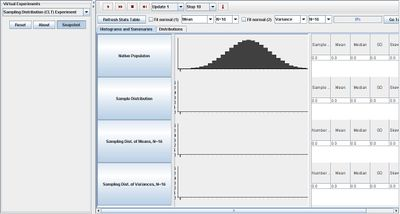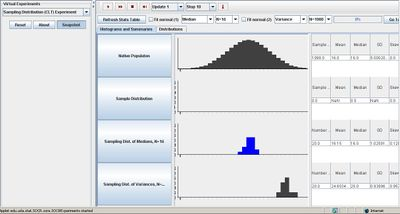# SOCR EduMaterials Activities SamplingDistributionCLTExperiment

## Description

The applet is part of the SOCR Experiments: Sampling Distribution (CLT) Experiment, which demonstrates the properties of the sampling distributions of various sample statistics. This applet can be used to demonstrate the Central Limit Theorem (CLT) as well as:

1. Investigate the effect of the Native population on various sample statistics 2. Study the effects of the sample-sizes on the sampling distribution 3. Determine which sample statistics may have known distributions (e.g. Normal), under what conditions 4. Explore the relations between Population parameters and their corresponding sample counterparts. For example, population mean/SD and sample mean/SD, symmetry, distribution shape, etc.

## Goal

The main focus of the Sampling Distribution (CLT) Experiment is to provide a simulation accessible to the public that demonstrates characteristics of various sample statistics and the Central Limit Theorem and to empirically demonstrate that the sample average is unique. Users should develop a better understanding of these two topics and be able to apply them to various types of activities as the concepts of a native distribution, sample, sample distribution, sampling distribution, parameter estimator, and data-driven numerical parameter estimate are reinforced.

## Experiment

Go to the SOCR Experiment SOCR Experiment and select the Sampling Distribution Experiment from the drop-down list of experiments on the top left. The image below shows the initial view of this experiment:When pressing the play button, one trial will be executed and recorded in the distribution table below. The fast forward button symbolizes the nth number of trials to be executed each time. The stop button ceases any activity and is helpful when the experimenter chooses “continuous,” indicating an infinite number of events. The fourth button will reset the entire experiment, deleting all previous information and data collected. The “update” scroll indicates nth number of trials (1, 10, 100, or 1000) performed when selecting the fast forward button and the “stop” scroll indicates the maximum number of trials in the experiment.

As the number of trials increase, notice that the graphs of the sampling distribution of medians and sampling distribution of variances converge to a normal curve. When the value N for variances increases, this graph shifts to the right. The image below demonstrates this:Under the distribution page, the user has the ability to select the specific experiment in the SOCR list of experiments and utilize it in the native population to determine the figures for the sampling distribution of means and variances. The figure below illustrates this ability when selecting the Beta distribution:

Error creating thumbnail: File missing

## Applications

The Sampling Distribution Experiment is a simple application that can be used in many different types of situations such as:

Suppose researchers want to determine the effects of asymmetry of the native distribution on the Central Limit Theorem. By using this application, they are able to determine the results from asymmetry as well as gaps and continuity.

Suppose students want to be able to understand when they can expect events to have Central Limit Theorem properties. This simulation will assist their needs by manipulating and implementing the parameters of the experiments and relate them to specific events.